×

Search anything:

# Circular Queue / Ring Buffer / Circular Buffer

#### Data Structures queue circular queueGet this book -> Problems on Array: For Interviews and Competitive Programming

Reading time: 25 minutes | Coding time: 10 minutes

A Circular Queue is an extension of the Queue data structure such that the last element of the queue links to the first element. It is known as Ring Buffer, Circular Buffer or Cyclic Buffer.

A queue is a data structure used to store data randomly distributed over memory. Here is reference for Queue

In Linear Queue Data Structure, we have two pointers Front and Rear. Elements are inserted in Rear while the Front is maintained to remove the element in the dequeue process.

In a normal Queue, we can insert elements until queue becomes full. Once the queue is full, no additional elements can be added even after removing an element from Queue or if there is a space in front of queue.
The only way is to reset the linear queue is to reinitialize or a fresh start.That's where the Circular queue come in picture to overcome Linear Queue Data Structure.

### Definition

Circular Queue is also a linear data structure, which follows the principle of FIFO(First In First Out), but instead of ending the queue at the last position, it again starts from the first position after the last, hence making the queue behave like a circular data structure.It is also called ‘Ring Buffer’.

Circular Queue can be implemented using:

• Array

### Basic Operations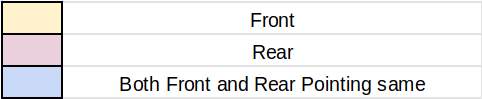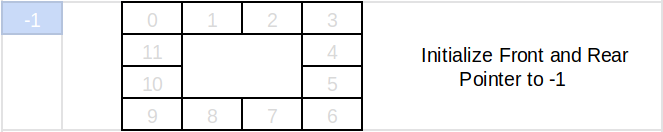INTIALIZATION

``````SIZE  = VALUE     // Size of Queue
ARRAY [SIZE]      // Array of Queue
FRONT = -1        // Initializing Front Pointer to -1
REAR  = -1        // Initializing Rear Pointer to -1
``````

Operation in Circular Queue are:

1. Front() : Function to get Front element of Circular Queue.
``````Front():
IF FRONT != -1 :
PRINT ARRAY[FRONT] // If FRONT !=-1 i.e. no. of elements != 0 then print the element at FRONT
ELSE :
PRINT "NO ELEMENTS IN QUEUE" // If circular queue is empty.
``````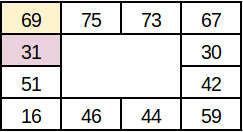On calling Front(), it will print 69
1. Rear() : Function to get Rear element of Circular Queue.
``````    Rear():
IF REAR != -1 :
PRINT ARRAY[REAR] // If REAR !=-1 i.e. no. of elements != 0 then print the element at REAR
ELSE :
PRINT "NO ELEMENTS IN QUEUE" // If circular queue is empty.
``````On calling Rear(), it will print 31
1. EnQueue(value) : Function to insert value at Rear position in Circular Queue.
``````EnQueue(value):
IF (REAR+1)%SIZE == FRONT :   // If circular queue is full.
PRINT "QUEUE IS FULL"
ELSE IF FRONT == -1 :         // If circular queue is empty.
FRONT = 0
REAR = 0
ARRAY[REAR] = value
ELSE :
REAR = REAR + 1
ARRAY[REAR] = value
``````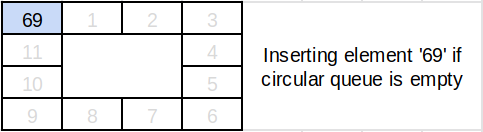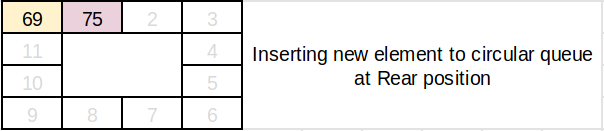1. DeQueue() : Function to remove element at Front position in Circular Queue.
``````DeQueue():
IF FRONT == -1  :             // If circular queue is empty.
PRINT "QUEUE IS EMPTY"
ELSE IF FRONT == REAR :         // If circular queue has only one element
TEMP = ARRAY[FRONT]
FRONT = -1
REAR = -1
RETURN TEMP
ELSE :
TEMP = ARRAY[FRONT]
FRONT = (FRONT + 1)%SIZE
RETURN TEMP
``````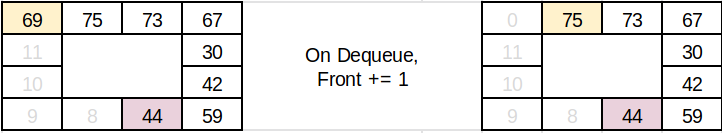1. Size() : Function to display size of Circular Queue.
``````Size():
IF FRONT == -1  :             // If circular queue is empty.
PRINT "QUEUE IS EMPTY"
ELSE IF FRONT == REAR :       // If circular queue has only one element.
RETURN 1
ELSE IF (REAR < FRONT):
RETURN (REAR + (SIZE - FRONT + 1))
ELSE :
RETURN (REAR - FRONT + 1)
``````
1. Display() : Function to display all elements in Circular Queue.
``````    Display():
IF FRONT == -1  :             // If circular queue is empty.
PRINT "QUEUE IS EMPTY"
ELSE IF FRONT == REAR :       // If circular queue has only one element.
PRINT ARRAY[FRONT]
ELSE IF (REAR < FRONT):
FOR I IN RANGE(FRONT,SIZE):
PRINT ARRAY[I]
FOR I IN RANGE(0,REAR):
PRINT ARRAY[I]
ELSE :
FOR I IN RANGE(FRONT,REAR):
PRINT ARRAY[I]
``````On calling Display(), it will print all elements in circular queue

### Complexity

• Time complexity of EnQueue(), DeQueue() operation is O(1) as there is no loop in any of the operation.
• Space complexity is O(n) where n is number of elements.

### Implementation

``````front = rear = -1     # global declaration of front and rear pointer
circular_queue = []   # Initializaiton of Circular Queue
size = int(input("Enter the Size of Circular Queue : "))

def init(size):
# initializing circular queue with none
return [None for i in range(size)]

def Front():
global front, rear, circular_queue
if (front == -1):
print('Circular Queue is Empty !!')
else:
print ("Element at Front : ",circular_queue[front])

def Rear():
global front, rear, circular_queue
if (rear == -1):
print('Circular Queue is Empty !!')
else:
print ("Element at Rear : ",circular_queue[rear])

def EnQueue(value):
global front, rear, circular_queue
if (front == -1):
front = 0
rear = 0
circular_queue[rear] = value
elif ((rear+1)%size == front):
print("Circular Queue is Full !!")
else :
# next position of rear
rear = (rear + 1)%size
circular_queue[rear] = value

def DeQueue():
global front, rear, circular_queue
if (rear == -1):
print('Circular Queue is Empty !!')
elif ( front==rear ):
print("Element Deleted : ",circular_queue[front])
front = -1
rear = -1
else :
print("Element Deleted : ",circular_queue[front])
front = (front + 1)%size

def Size():
global front, rear, circular_queue
if (front == -1):
print('Circular Queue is Empty !!')
elif ( front==rear ):
print('Size of Circular Queue : 1')
elif (rear<front) :
print('Size of Circular Queue : ',(rear + (size - front)))
else :
print('Size of Circular Queue : ',(rear - front + 1))

def Display():
global front, rear, circular_queue
if (front == -1):
print('Circular Queue is Empty !!')
elif (rear == front):
print("Circular Queue : ",circular_queue[front])
else :
if(rear < front):
print("Circular Queue : ",circular_queue[front:size]+circular_queue[:rear+1])
else :
print("Circular Queue : ",circular_queue[front:rear+1])
if ((rear+1)%size == front):
print("Circular Queue is Full !!")

circular_queue =  init(size)
print("After Initialization : ",circular_queue)

EnQueue(69)
EnQueue(75)
EnQueue(73)
EnQueue(67)
EnQueue(44)

Display()

Front()
Rear()
Size()

Display()

DeQueue()
DeQueue()

Display()
Size()

EnQueue(30)
EnQueue(51)

Front()
Rear()

Display()

EnQueue(31)

# This code is contributed by Vedant Wakalkar
``````

### Output

``````Enter the Size of Circular Queue : 5
After Initialization :  [None, None, None, None, None]

Circular Queue :  [69, 75, 73, 67, 44]
Circular Queue is Full !!

Element at Front :  69
Element at Rear :  44
Size of Circular Queue :  5

Circular Queue :  [69, 75, 73, 67, 44]
Circular Queue is Full !!

Element Deleted :  69
Element Deleted :  75

Circular Queue :  [73, 67, 44]
Size of Circular Queue :  3

Element at Front :  73
Element at Rear :  51

Circular Queue :  [73, 67, 44, 30, 51]
Circular Queue is Full !!

Circular Queue is Full !!
``````

### Application

• In CPU scheduling viz Round Robin Scheduling.
• Memory Management : unused memory locations can be utilized using circular queue.
• Computer controlled Traffic Signal System uses circular queue.

## Question

#### Circular queue is initialized,

Following operations are performed
EnQueue(54)
EnQueue(23)
EnQueue(12)
DeQueue()
EnQueue(86)
What will be Element at Front?
23
54
12
86
Initially []
EnQueue(54) 
EnQueue(23) [54,23]
EnQueue(12) [54,23,12]
DeQueue() [23,12]
EnQueue(86) [23,12,86]